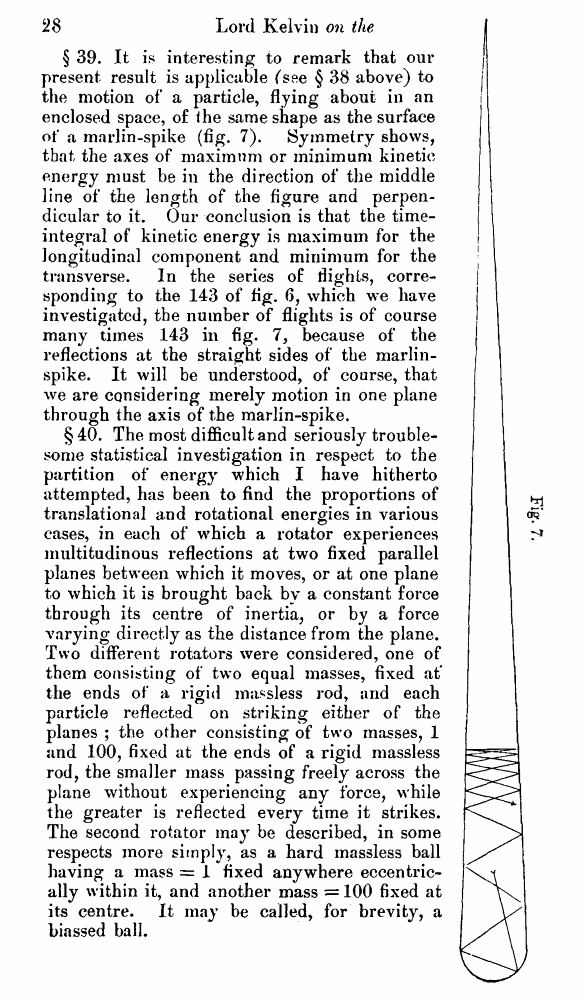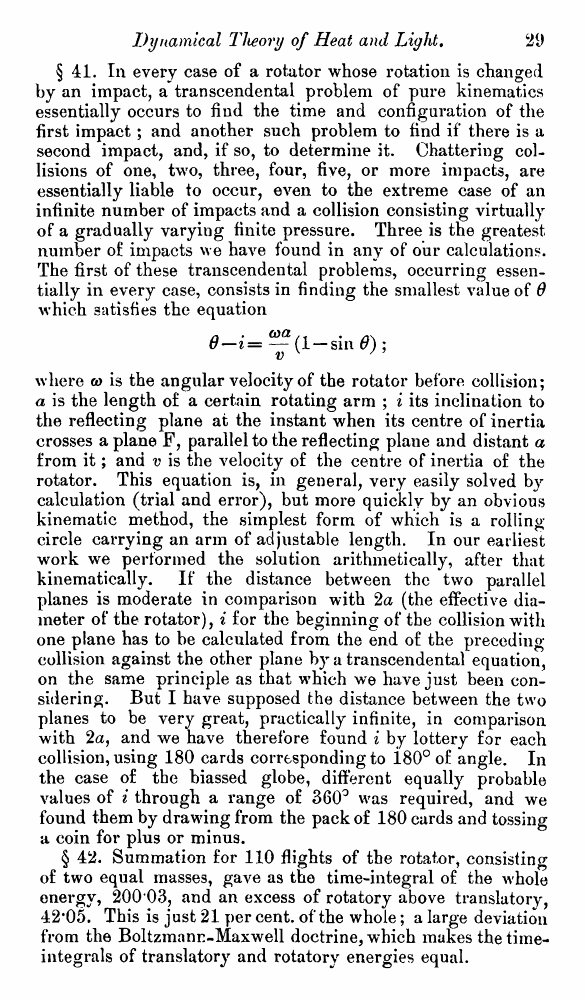﻿ Lord Kelvin. Nineteenth-Century Clouds over the Dynamical Theory of Heat and Light. // Phil. Mag. S. 6. Vol. 2. No. 7. July 1901.

# Lord Kelvin. Nineteenth-Century Clouds over the Dynamical Theory of Heat and Light. // Phil. Mag. S. 6. Vol. 2. No. 7. July 1901.

В начало   Другие форматы   <<<     Страница 28   >>>1  2  3  4  5  6  7  8  9  10  11  12  13  14  15  16  17  18  19  20  21  22  23  24  25  26  27  28 29  30  31  32  33  34  35  36  37  38  39  40 § 39. It is interesting to remark that our present result is applicable (see § 38 above) to the motion of a particle, flying about in an enclosed space, of the same shape as the surface of a inarlin-spike (fig. 7). Symmetry shows, that the axes of maximum or minimum kinetic energy must be in the direction of the middle line of the length of the figure and perpendicular to it. Our conclusion is that the time-integral of kinetic energy is maximum for the longitudinal component and minimum for the transverse. In the series of flights, corresponding to the 143 of fig. 6, which we have investigated, the number of flights is of course many times 143 in fig. 7, because of the reflections at the straight sides of the marlin-spike. It will be understood, of course, that we are considering merely motion in one plane through the axis of the marlin-spike. § 40. The most difficult and seriously troublesome statistical investigation in respect to the partition of energy which I have hitherto attempted, has been to find the proportions of translational and rotational energies in various cases, in each of which a rotator experiences multitudinous reflections at two fixed parallel planes between which it moves, or at one plane to which it is brought back by a constant force through its centre of inertia, or by a force varying directly as the distance from the plane. Two different rotators were considered, one of them consisting of two equal masses, fixed at* the ends of a rigid massless rod, and each particle reflected on striking either of the planes ; the other consisting of two masses, 1 and 100, fixed at the ends of a rigid massless rod, the smaller mass passing freely across the plane without experiencing any force, while the greater is reflected every time it strikes. The second rotator may be described, in some respects more simply, as a hard massless ball having a mass = 1 fixed anywhere eccentrically within it, and another mass = 100 fixed at its centre. It may be called, for brevity, a biassed ball. § 41. In every case of a rotator whose rotation is changed by an impact, a transcendental problem of pure kinematics essentially occurs to find the time and configuration of the first impact ; and another such problem to find if there is a second impact, and, if so, to determine it. Chattering collisions of one, twro, three, four, five, or more impacts, are essentially liable to occur, even to the extreme case of an infinite number of impacts and a collision consisting virtually of a gradually varying finite pressure. Three is the greatest number of impacts we have found in any of our calculations. The first of these transcendental problems, occurring essentially in every case, consists in finding the smallest value of 6 which satisfies the equation 6—i= ^(1 — sin 6) ; where co is the angular velocity of the rotator before collision; a is the length of a certain rotating arm ; i its inclination to the reflecting plane at the instant when its centre of inertia crosses a plane F, parallel to the reflecting plane and distant a from it; and v is the velocity of the centre of inertia of the rotator. This equation is, in general, very easily solved by calculation (trial and error), but more quickly by an obvious kinematic method, the simplest form of which is a rolling circle carrying an arm of adjustable length. In our earliest work we performed the solution arithmetically, after that kinematically. If the distance between the two parallel planes is moderate in comparison with 2a (the effective diameter of the rotator), i for the beginning of the collision with one plane has to be calculated from the end of the preceding collision against the other plane by a transcendental equation, on the same principle as that which we have just been considering. But I have supposed the distance between the two planes to be very great, practically infinite, in comparison with 2a, and we have therefore found i by lottery for each collision, using 180 cards corresponding to i80° of angle. In the case of the biassed globe, different equally probable values of i through a range of 360° was required, and we found them by drawing from the pack of 180 cards and tossing a coin for plus or minus. § 42. Summation for 110 flights of the rotator, consisting of two equal masses, gave as the time-integral of the whole energy, 200 03, and an excess of rotatory above translatory, 42*05. This is just 21 per cent, of the whole; a large deviation from the Boltzmann-Maxwell doctrine, which makes the time-integrals of translatory and rotatory energies equal.